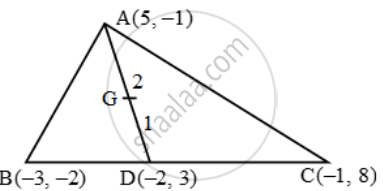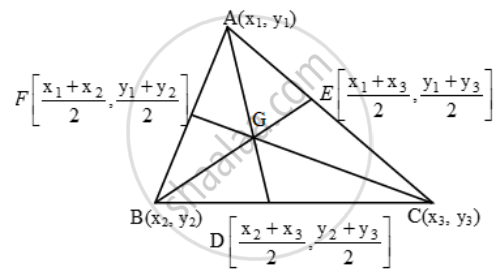Share

# If A (5, –1), B(–3, –2) and C(–1, 8) are the vertices of triangle ABC, find the length of median through A and the coordinates of the centroid. - CBSE Class 10 - Mathematics

#### Question

If A (5, –1), B(–3, –2) and C(–1, 8) are the vertices of triangle ABC, find the length of median through A and the coordinates of the centroid.

#### Solution

Let AD be the median through the vertex A of ∆ABC. Then, D is the mid-point of BC. So, the coordinates of

( \frac{-3-1}{2},\ \frac{-2+8}{2})

\therefore AD=sqrt((5+2)^{2}+(-1-3)^{2})=\sqrt{49+16}=\sqrt{65)Let G be the centroid of ∆ABC. Then, G lies on median AD and divides it in the ratio 2 : 1. So, coordinates of G are

( \frac{2\times (-2)+1\times 5}{2+1},\ \frac{2\times 3+1\times (-1)}{2+1})

=( \frac{-4+5}{3},\ \frac{6-1}{3})= ( \frac{1}{3},\frac{5}{3})

Application Of Section FormulaThe coordinates of the centroid of the triangle whose vertices are (x_1 , y_1 ), (x_2 , y_2 )

( (x_1+x_2+x_3)/3,(y_1+y_2+y_3)/{3})

Is there an error in this question or solution?

#### Video TutorialsVIEW ALL 

Solution If A (5, –1), B(–3, –2) and C(–1, 8) are the vertices of triangle ABC, find the length of median through A and the coordinates of the centroid. Concept: Section Formula.
S